Howdy, Stranger!

It looks like you're new here. If you want to get involved, click one of these buttons!

Options

Exercise 3 - Chapter 1

edited April 2018

1) Write down all the partitions of a two element set {$$\bullet,\ast$$}, order them as above, and draw the Hasse diagram.

2) Now do the same thing for a four element-set, say {1,2,3,4}. There should be 15 partitions.

Choose any two systems in your 15-element Hasse diagram, call them $$A$$ and $$B$$.

3) What is $$A\vee B$$, using the definition given in the paragraph above

4) Is it true that $$A\leq (A\vee B)$$ and $$B\leq (A\vee B)$$?

5) What are all the partitions $$C$$ for which both $$A\leq C$$ and $$B\leq C$$.

6) Is it true that in each case, $$(A\vee B)\leq C$$?

• Options
1.
edited April 2018

Here are some hints. Spoiler alert: I'll do part 2 of this exercise.

A partition of a set is a way of writing it as a disjoint union of nonempty subsets. These subsets are called blocks. Here are all 52 partitions of a 5-element set, as drawn by Watchduck on Wikicommons:We say one partition $$P$$ of a set is finer than another partition $$P'$$ if every block of $$P'$$ is a union of blocks of $$P$$. One could say "finer than or equal to", but people say just "finer". In this situation we also say $$P'$$ is coarser than $$P$$.

If $$P$$ is finer than $$P'$$, Fong and Spivak write $$P \le P'$$ . This is a somewhat arbitrary choice: we could have done it the other way. This relation $$\le$$ makes the collection of all partitions of a set $$S$$ into a poset, which is usually called $$\Pi(S)$$. This poset has a lot of interesting properties.

Here's a picture of all 15 partitions of a 4-element set, with finer ones nearer the bottom, and edges pointing from each partition to the next finer ones. This way of drawing a poset is called a Hasse diagram: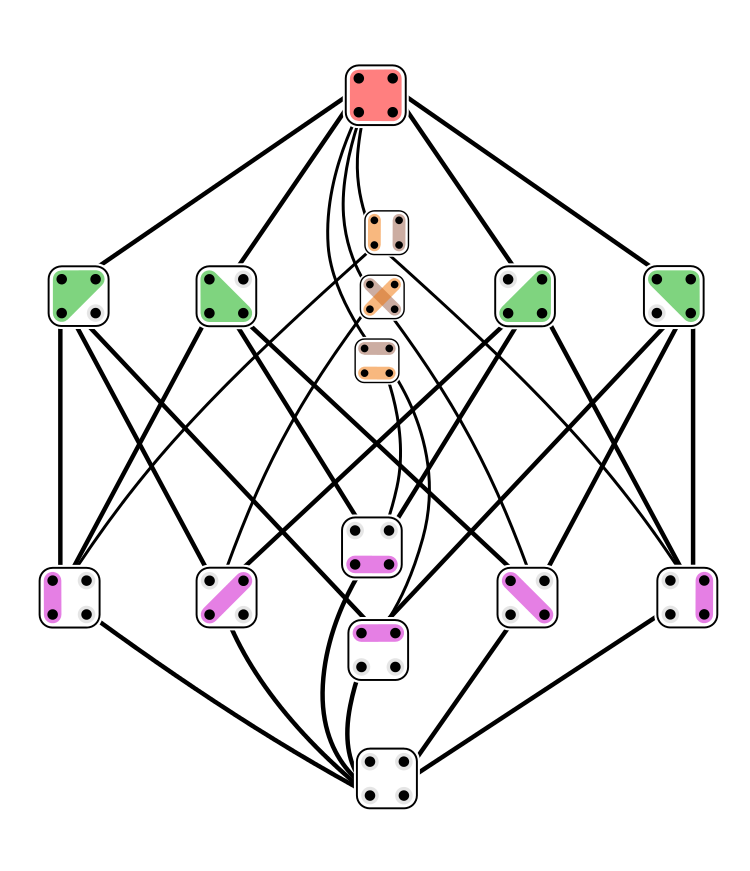The picture was drawn by Tilman Piesk on Wikicommons. Click on it for a lot more information.

For any two partitions $$P,P'$$ of a set $$S$$, there is a unique finest partition $$P \vee P'$$ that is coarser than both. In other words:

1. $$P \le P \vee P'$$ and $$P' \le P \vee P'$$.

2. If $$Q$$ is any partition with $$P \le Q$$ and $$P' \le Q$$, then $$P \vee P' \le Q$$.

This is called the join of $$P$$ and $$P'$$.

There's also a unique coarsest partition $$P \wedge P'$$ that is finer than both $$P$$ and $$P'$$. This is called the meet of $$P$$ and $$P'$$.

Comment Source:Here are some hints. Spoiler alert: I'll do part 2 of this exercise. A **[partition](https://en.wikipedia.org/wiki/Partition_of_a_set)** of a set is a way of writing it as a disjoint union of nonempty subsets. These subsets are called **blocks**. Here are all 52 partitions of a 5-element set, as drawn by [Watchduck](https://commons.wikimedia.org/wiki/File:Set_partitions_5;_circles.svg) on Wikicommons: <img src = "https://upload.wikimedia.org/wikipedia/commons/thumb/1/19/Set_partitions_5%3B_circles.svg/500px-Set_partitions_5%3B_circles.svg.png"> We say one partition \$$P\$$ of a set is **finer** than another partition \$$P'\$$ if every block of \$$P'\$$ is a union of blocks of \$$P\$$. One could say "finer than or equal to", but people say just "finer". In this situation we also say \$$P'\$$ is **coarser** than \$$P\$$. If \$$P\$$ is finer than \$$P'\$$, Fong and Spivak write \$$P \le P'\$$ . This is a somewhat arbitrary choice: we could have done it the other way. This relation \$$\le\$$ makes the collection of all partitions of a set \$$S\$$ into a poset, which is usually called \$$\Pi(S)\$$. This poset has a lot of interesting properties. Here's a picture of all 15 partitions of a 4-element set, with finer ones nearer the bottom, and edges pointing from each partition to the next finer ones. This way of drawing a poset is called a [Hasse diagram](https://en.wikipedia.org/wiki/Hasse_diagram): <a href = "https://blogs.ams.org/visualinsight/2015/06/15/lattice-of-partitions/"> <img width = "500" src = "https://blogs.ams.org/visualinsight/files/2015/06/lattice_of_partitions.png"> </a> The picture was drawn by [Tilman Piesk](https://commons.wikimedia.org/wiki/File:Set_partitions_4;_Hasse;_circles.svg) on Wikicommons. Click on it for a lot more information. For any two partitions \$$P,P'\$$ of a set \$$S\$$, there is a unique finest partition \$$P \vee P'\$$ that is coarser than both. In other words: 1. \$$P \le P \vee P'\$$ and \$$P' \le P \vee P'\$$. 2. If \$$Q\$$ is any partition with \$$P \le Q\$$ and \$$P' \le Q\$$, then \$$P \vee P' \le Q\$$. This is called the **join** of \$$P\$$ and \$$P'\$$. There's also a unique coarsest partition \$$P \wedge P'\$$ that is finer than both \$$P\$$ and \$$P'\$$. This is called the **meet** of \$$P\$$ and \$$P'\$$.
• Options
2.
edited April 2018

3) What is A∨B, using the definition given in the paragraph above

I am choosing A and B as dented on the picture belowSo A∨B is a subset that has a union of blocks in A and B (hope this wording is correct).

Therefore, it is4) Is it true that A≤(A∨B) and B≤(A∨B) ?

I think this is true. The effects of the ∨-operation are 'additive' and cannot be destructive. And, because ≤ - relation is defined as 'whatever is connected in A, should remain connected in (A∨B) , we cannot have a situation where there is a connection in A, but not in (A∨B).

5) What are all the partitions C for which both A≤C and B≤C

In my case (because of how I chose A and B), it is6) Is it true that in each case, (A∨B)≤C ?

It is not true in general. Although this happened to be true in my selection example. It is not true in general, because ∨ can produce generative effects, such as new connections, that were not part of C , given how C could be selected according to 5).

Comment Source:> 3) What is A∨B, using the definition given in the paragraph above I am choosing A and B as dented on the picture below <a href="http://imgbb.com/"><img src="http://image.ibb.co/iQFZFS/Ex3_Chapter1.png" alt="Ex3_Chapter1" border="0"></a> So A∨B is a subset that has a union of blocks in A and B (hope this wording is correct). Therefore, it is <a href="http://imgbb.com/"><img src="http://image.ibb.co/nE7WvS/Ex3_Chapter1_p2.png" alt="Ex3_Chapter1_p2" border="0"></a> > 4) Is it true that A≤(A∨B) and B≤(A∨B) ? I think this is true. The effects of the ∨-operation are 'additive' and cannot be destructive. And, because ≤ - relation is defined as 'whatever is connected in A, should remain connected in (A∨B) , we cannot have a situation where there is a connection in A, but not in (A∨B). > 5) What are all the partitions C for which both A≤C and B≤C In my case (because of how I chose A and B), it is <a href="http://imgbb.com/"><img src="http://image.ibb.co/nE7WvS/Ex3_Chapter1_p2.png" alt="Ex3_Chapter1_p2" border="0"></a> > 6) Is it true that in each case, (A∨B)≤C ? It is not true in general. Although this happened to be true in my selection example. It is not true in general, because ∨ can produce generative effects, such as new connections, that were not part of C , given how C could be selected according to 5).
• Options
3.
edited April 2018

Okay, I will do the easy one!

1. There are exactly two partitions of a two-element set {$$\bullet,\ast$$}, viz. {{$$\bullet$$}, {$$\ast$$}}, and {{$$\bullet,\ast$$}}. The first partition is finer than the second, from which we can draw the corresponding Hasse diagram in the obvious way.
Comment Source:Okay, I will do the easy one! 1. There are exactly two partitions of a two-element set {\$$\\bullet,\ast\$$}, _viz._ {{\$$\bullet\$$}, {\$$\ast\$$}}, and {{\$$\\bullet,\ast\$$}}. The first partition is finer than the second, from which we can draw the corresponding Hasse diagram in the obvious way.
• Options
4.
edited April 2018

Thanks, Vishul! A really annoying fact about MathJax on this website is that braces don't work:

\$$\{x,y\} \$$

delivers

$${x,y}$$

So, I retyped your comment using a hack. This was a bit tiring given the nested braces! I typed

There are exactly two partitions of the two-element set {\$$\bullet,\ast\$$}, _viz._ {{\$$\bullet, \ast\$$}}, and {{\$$\bullet\$$}, {\$$\ast\$$}}.

and got

There are exactly two partitions of the two-element set {$$\bullet,\ast$$}, viz. {{$$\bullet, \ast$$}}, and {{$$\bullet$$}, {$$\ast$$}}.

I'm glad we're doing category theory and not set theory!

Comment Source:Thanks, Vishul! A really annoying fact about MathJax on this website is that braces don't work: \$$\{x,y\} \$$ delivers \$$\{x,y\} \$$ So, I retyped your comment using a hack. This was a bit tiring given the nested braces! I typed > There are exactly two partitions of the two-element set {\$$\bullet,\ast\$$}, _viz._ > {{\$$\bullet, \ast\$$}}, and {{\$$\bullet\$$}, {\$$\ast\$$}}. and got > There are exactly two partitions of the two-element set {\$$\bullet,\ast\$$}, _viz._ {{\$$\bullet, \ast\$$}}, and {{\$$\bullet\$$}, {\$$\ast\$$}}. I'm glad we're doing category theory and not set theory!
• Options
5.

Thanks, John! I did figure out after a few tries that braces indeed do not work in the "LaTeX-way" on this site, at least when commenting :) And, I did figure out the hack you provided. Thanks, again.

Comment Source:Thanks, John! I did figure out after a few tries that braces indeed do not work in the "LaTeX-way" on this site, at least when commenting :) And, I did figure out the hack you provided. Thanks, again.
• Options
6.
edited April 2018

Curly braces can be used via \$$A = \\{x,y,z\\}\$$: $$A = \{ x,y,z \}$$

It's annoying, but you need two backslashes before the braces.

\$$\begin{cases} 1 \\\\ 4 \end{cases}\$$

$$\begin{cases} 1 \\ 4 \end{cases}$$

There's a similar issue when you want a newline embedded in the text, like trying to use cases above.

Comment Source:Curly braces can be used via \$$A = \\{x,y,z\\}\$$: \$$A = \\{ x,y,z \\}\$$ It's annoying, but you need two backslashes before the braces. \$$\begin{cases} 1 \\\\ 4 \end{cases}\$$ \$$\begin{cases} 1 \\\\ 4 \end{cases}\$$ There's a similar issue when you want a newline embedded in the text, like trying to use cases above.
• Options
7.

Thanks for explaining how it works, Jared.

Comment Source:Thanks for explaining how it works, Jared.
• Options
8.
edited April 2018

Vladislav Papayan #2 wrote:

6) Is it true that in each case, $$(A \vee B)\leq C$$?

It is not true in general. Although this happened to be true in my selection example. It is not true in general, because $$\vee$$ can produce generative effects, such as new connections, that were not part of $$C$$, given how $$C$$ could be selected according to 5.

Is this right? I thought that by definition $$(A \vee B) \le C$$ if both $$A \le C$$ and $$B \le C$$ (which are the criteria for selecting $$C$$, stated in part 5 of the exercise).

Comment Source:Vladislav Papayan #2 wrote: > > 6) Is it true that in each case, \$$(A \vee B)\leq C\$$? > It is not true in general. Although this happened to be true in my selection example. It is not true in general, because \$$\vee\$$ can produce generative effects, such as new connections, that were not part of \$$C\$$, given how \$$C\$$ could be selected according to 5. Is this right? I thought that by definition \$$(A \vee B) \le C \$$ if both \$$A \le C \$$ and \$$B \le C \$$ (which are the criteria for selecting \$$C\$$, stated in part 5 of the exercise).
• Options
9.
edited April 2018

Dan #8 you are right, for Hasse diagrams at least this property always holds. Where the book talks about generative effects (1.1.) it also says this holds for any C but I don't know if there is some other context where the Join fails to keep (A ∨ B) ≤ C when A ≤ C and B ≤ C.

If there was a C such that C ≤ (A ∨ B) then there would be an element in either A or B that isn't in C violating A ≤ C and B ≤ C.

• The reason I'm not sure is because my argument hinges on the concept of an element which is not necessarily something I have access to when reasoning about categories?
Comment Source:Dan #8 you are right, for Hasse diagrams at least this property always holds. Where the book talks about generative effects (1.1.) it also says this holds for any C but I don't know if there is some other context where the Join fails to keep (A ∨ B) ≤ C when A ≤ C and B ≤ C. If there was a C such that C ≤ (A ∨ B) then there would be an element in either A or B that isn't in C violating A ≤ C and B ≤ C. * The reason I'm not sure is because my argument hinges on the concept of an element which is not necessarily something I have access to when reasoning about categories?
• Options
10.

Ilmu Khan #9 - Let's try using the definition of $$\vee$$. By definition, $$A \vee B$$ is the least (=smallest) element $$X$$ such that $$A \le X$$ and $$B \le X$$. So question 6) is asking us this:

Supopose $$A \le C$$ and $$B \le C$$ and $$X$$ is the smallest element such that $$A \le X$$ and $$B \le X$$. Does this imply $$X \le C$$?

Comment Source:Ilmu Khan #9 - Let's try using the definition of \$$\vee\$$. By definition, \$$A \vee B\$$ is the least (=smallest) element \$$X\$$ such that \$$A \le X\$$ and \$$B \le X\$$. So question 6) is asking us this: > Supopose \$$A \le C\$$ and \$$B \le C\$$ and \$$X\$$ is the smallest element such that \$$A \le X\$$ and \$$B \le X\$$. Does this imply \$$X \le C\$$?
• Options
11.
edited April 2018

Another attempt to summarise things with pictures and informal description. This time: Partitions. Comments/suggests greatfully accepted as before.

Partitions are covered in section 1.2.1 of the book. They're also covered in Exercise 3.

What is a Partition?

John describes it as follows in this comment:

A partition of a set is a way of writing it as a disjoint union of nonempty subsets.

That's pretty accessible, even for non-mathematicians like me. No harm in illustrating with an example though. Let's take a set of colleagues in some fictitious workplace: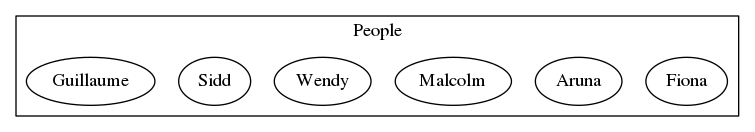And create a partition based on their roles: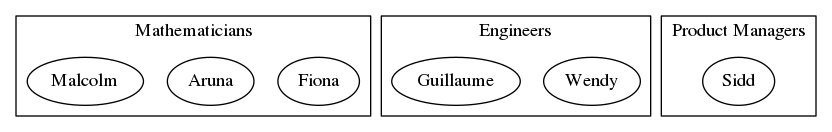What makes that a partition? Let's go back to the definition:

a disjoint union of non-empty subsets

• Each of Product Managers, Engineers and Mathematicians has at least one person in it. So there are no empty subsets.
• Each person fits in exactly one subset. That means:
• The subsets aren't overlapping, they're disjoint.
• the original People set can be constructed by joining together the three subsets. In set theory, "union" just means "join together".

Here's a slightly different way of defining a partition, drawing on some of the language above.

A partition of a set splits the members into separate subgroups such that:

1. Every member of the set is contained in exactly one subgroup, and
2. Every subgroup contains at least one member

What about the definition in the book?

Partitions are introduced in definition 1.8 in the book:

If $$A$$ is a set, a partition of $$A$$ consists of a set $$P$$ and, for each $$p \in P$$, a non-empty subset $$A_p\subset A$$, such that:

$$A=\bigcup_{p\in P}A_p \qquad\text{and}\qquad \text{if }p\neq q\text{ then }A_P\cap A_Q=\emptyset$$ There's a bit more in there, so let's break it down. First, it introduces a new set $$P$$ with members $$p$$. This just describes the partition itself, where each subgroup (or part) is a member of P. In our example above, P is the set of roles, as follows: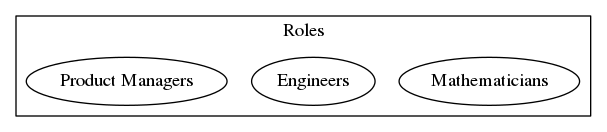We now have the set $$A$$ being partitioned, and the set $$P$$ defining the parts. Picking up the next part of the definition:

for each $$p \in P$$, a non-empty subset $$A_p\subset A$$

So: each $$p$$ - each part - maps to a non-empty subset of $$A$$. That's just what we drew in the partition diagram above. Here's a slightly different way of drawing it, such that $$A$$ and $$P$$ are both shown consistently as sets: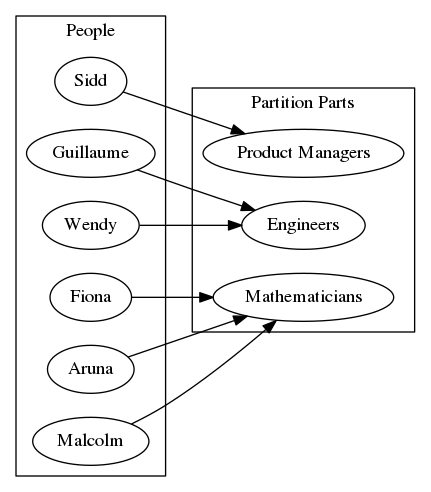We can now enumerate the subsets $$A_p \subset A$$:

• $$A_{Product Managers} = (Sidd)$$
• $$A_{Mathematicians} = (Fiona, Aruna, Malcolm)$$
• $$A_{Engineers} = (Guillaume, Wendy)$$

Back to the definition:

$$A=\bigcup_{p\in P}A_p$$ Reading this in natural language, it says $$A$$ is the union $$\bigcup$$ of all subsets $$A_p$$ for each $$p\in P$$. So $$A = A_{ProductManagers} + A_{Mathematicians} + A_{Engineers}$$.

And so the final component of the definition:

$$\text{if }p\neq q\text{ then }A_P\cap A_Q=\emptyset$$ This is the 'disjoint', or non-overlapping clause. Translating that literally into words:

If p isn't equal to q, then the intersection of subsets $$A_P$$ and $$A_Q$$ is the empty set.

Less prosaically, $$A_P$$ and $$A_Q$$ don't share any members unless $$p = q$$ (in which case $$p$$ and $$q$$ represent the same element).

We're back to where we started now. $$P$$ is a partition of $$A$$ because:

1. $$P$$ comprises one or more parts
2. Each part of $$P$$ contains at least one element of $$A$$
3. Each element of $$A$$ is contained in exactly one part of $$P$$

Comparing Partitions

So far we've only looked at one partition of our People set. Here's another: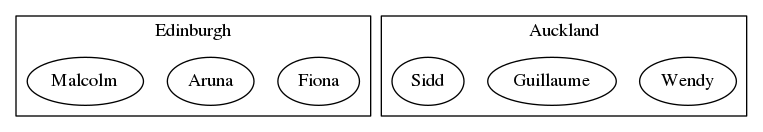This partitions based on location as opposed to role. It only has 2 parts where the role-based partition had 3. We can compare the two partions as follows:

• The Role partition is finer than the Location partition
• The Location partition is coarser than the Role partition

These statements are inverses. Formally, a partition $$P_1$$ is finer than $$P_2$$ if:

1. $$P_1$$ and $$P_2$$ partition the same set, and
2. Every part of $$P_2$$ is a union of parts in $$P_1$$

Relating that to the Location and Role Partitions:

1. Auckland is the union of Engineers and Product Managers
2. Edinburgh is the same as Mathematicians.

But wait: surely Edinburgh isn't a union of Location parts? Technically it is: Edinburgh is the union of Mathematicians and the empty set $$\emptyset$$. The empty set is a subset of every set by definition.

A counter example

It's perhaps tempting to define the finer/coarser relation as simply:

• A finer partition has more parts

However, that doesn't work. Suppose Guillaume moved from Auckland to Edinburgh: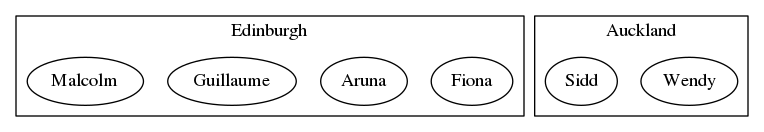We can't now relate the revised Location partition with the Role-based alternative. There's no way to combine the Product Manager, Engineer and Mathematician parts, using the union operator, to produce the Edinburgh and Auckland parts.

Comment Source:Another attempt to summarise things with pictures and informal description. This time: Partitions. Comments/suggests greatfully accepted as before. Partitions are covered in section 1.2.1 of [the book](https://arxiv.org/pdf/1803.05316.pdf). They're also covered in [Exercise 3](https://forum.azimuthproject.org/discussion/1876/exercise-3-chapter-1#latest). ## What is a Partition? John describes it as follows in [this comment](https://forum.azimuthproject.org/discussion/comment/16368/#Comment_16368): > A partition of a set is a way of writing it as a disjoint union of nonempty subsets. That's pretty accessible, even for non-mathematicians like me. No harm in illustrating with an example though. Let's take a set of colleagues in some fictitious workplace: ![set of people](https://raw.githubusercontent.com/sfinnie/CategoryTheoryCourseNotes/master/partitions/img/people.png) And create a partition based on their roles: ![set of people partitioned by role](https://raw.githubusercontent.com/sfinnie/CategoryTheoryCourseNotes/master/partitions/img/people-roles.png) What makes that a partition? Let's go back to the definition: > a disjoint union of non-empty subsets * Each of *Product Managers*, *Engineers* and *Mathematicians* has at least one person in it. So there are no empty subsets. * Each person fits in exactly one subset. That means: * The subsets aren't overlapping, they're *disjoint*. * the original People set can be constructed by joining together the three subsets. In set theory, "union" just means "join together". Here's a slightly different way of defining a partition, drawing on some of the language above. > A partition of a set splits the members into separate subgroups such that: > 1. Every member of the set is contained in exactly one subgroup, and > 1. Every subgroup contains at least one member ### What about the definition in the book? Partitions are introduced in definition 1.8 in the book: > If \$$A\$$ is a set, a *partition* of \$$A\$$ consists of a set \$$P\$$ and, for each \$$p \in P\$$, a non-empty subset \$$A_p\subset A\$$, such that: $$A=\bigcup_{p\in P}A_p \qquad\text{and}\qquad \text{if }p\neq q\text{ then }A_P\cap A_Q=\emptyset$$ There's a bit more in there, so let's break it down. First, it introduces a new set \$$P\$$ with members \$$p\$$. This just describes the partition itself, where each subgroup (or *part*) is a member of P. In our example above, P is the set of roles, as follows: ![roles](https://raw.githubusercontent.com/sfinnie/CategoryTheoryCourseNotes/master/partitions/img/roles.png) We now have the set \$$A\$$ being partitioned, and the set \$$P\$$ defining the parts. Picking up the next part of the definition: > for each \$$p \in P\$$, a non-empty subset \$$A_p\subset A\$$ So: each \$$p\$$ - each *part* - maps to a non-empty subset of \$$A\$$. That's just what we drew in the partition diagram above. Here's a slightly different way of drawing it, such that \$$A\$$ and \$$P\$$ are both shown consistently as sets: ![Mapping of people to roles](https://raw.githubusercontent.com/sfinnie/CategoryTheoryCourseNotes/master/partitions/img/people-roles-sets.png) We can now enumerate the subsets \$$A_p \subset A\$$: * \$$A_{Product Managers} = (Sidd)\$$ * \$$A_{Mathematicians} = (Fiona, Aruna, Malcolm)\$$ * \$$A_{Engineers} = (Guillaume, Wendy)\$$ Back to the definition: $$A=\bigcup_{p\in P}A_p$$ Reading this in natural language, it says \$$A\$$ is the union \$$\bigcup\$$ of all subsets \$$A_p\$$ for each \$$p\in P \$$. So \$$A = A_{ProductManagers} + A_{Mathematicians} + A_{Engineers}\$$. And so the final component of the definition: $$\text{if }p\neq q\text{ then }A_P\cap A_Q=\emptyset$$ This is the 'disjoint', or non-overlapping clause. Translating that literally into words: > If p isn't equal to q, then the intersection of subsets \$$A_P\$$ and \$$A_Q\$$ is the empty set. Less prosaically, \$$A_P\$$ and \$$A_Q\$$ don't share any members unless \$$p = q\$$ (in which case \$$p\$$ and \$$q\$$ represent the same element). We're back to where we started now. \$$P\$$ is a partition of \$$A\$$ because: 1. \$$P\$$ comprises one or more parts 2. Each part of \$$P\$$ contains at least one element of \$$A\$$ 3. Each element of \$$A\$$ is contained in exactly one part of \$$P\$$ ## Comparing Partitions So far we've only looked at one partition of our People set. Here's another: ![Mapping of people to roles](https://raw.githubusercontent.com/sfinnie/CategoryTheoryCourseNotes/master/partitions/img/people-locations.png) This partitions based on location as opposed to role. It only has 2 parts where the role-based partition had 3. We can compare the two partions as follows: * The Role partition is ***finer*** than the Location partition * The Location partition is ***coarser*** than the Role partition These statements are inverses. Formally, a partition \$$P_1\$$ is finer than \$$P_2\$$ if: 1. \$$P_1\$$ and \$$P_2\$$ partition the same set, and 2. Every part of \$$P_2\$$ is a union of parts in \$$P_1\$$ Relating that to the Location and Role Partitions: 1. *Auckland* is the union of *Engineers* and *Product Managers* 2. *Edinburgh* is the same as *Mathematicians*. But wait: surely Edinburgh isn't a union of Location parts? Technically it is: *Edinburgh* is the union of *Mathematicians* and the empty set \$$\emptyset\$$. The empty set is a subset of every set by definition. ### A counter example It's perhaps tempting to define the finer/coarser relation as simply: * A finer partition has more parts However, that doesn't work. Suppose Guillaume moved from Auckland to Edinburgh: ![Mapping of people to roles](https://raw.githubusercontent.com/sfinnie/CategoryTheoryCourseNotes/master/partitions/img/people-locations2.png) We can't now relate the revised Location partition with the Role-based alternative. There's no way to combine the *Product Manager*, *Engineer* and *Mathematician* parts, using the union operator, to produce the *Edinburgh* and *Auckland* parts.
• Options
12.
edited April 2018

Is the only difference between a partition and a topology that the empty set is not part of the partition and that the member sets of the partition are disjoint?

Comment Source:Is the only difference between a partition and a topology that the empty set is not part of the partition and that the member sets of the partition are disjoint?
• Options
13.
edited April 2018

John Baez #10 Thanks for the explanation. Since $$X$$ is the smallest element such that $$A \le X$$ and $$B \le X$$. It follows that any $$C$$ for which $$A \le C$$ and $$B \le C$$, $$\implies$$ $$C \geq X$$, i.e. $$C$$ is at least the same as $$X$$ or is the union of $$X$$ and another set.

Comment Source:John Baez #10 Thanks for the explanation. Since \$$X\$$ is the smallest element such that \$$A \le X\$$ and \$$B \le X\$$. It follows that any \$$C\$$ for which \$$A \le C\$$ and \$$B \le C\$$, \$$\implies\$$ \$$C \geq X\$$, i.e. \$$C\$$ is at least the same as \$$X\$$ or is the union of \$$X\$$ and another set.
• Options
14.

Is the only difference between a partition and a topology that the empty set is not part of the partition and that the member sets of the partition are disjoint?

No, there are other differences. Check out the definition of a topology. They're so different that I'd rather not talk about topology here.

Comment Source:[Jared Davis wrote](https://forum.azimuthproject.org/discussion/comment/16828/#Comment_16828): > Is the only difference between a partition and a topology that the empty set is not part of the partition and that the member sets of the partition are disjoint? No, there are other differences. Check out [the definition of a topology](https://en.wikipedia.org/wiki/Topology#Topologies_on_sets). They're so different that I'd rather not talk about topology here.
• Options
15.
edited April 2018

Scott Finnie: thanks for another nice pictorial explanation!

You wrote:

Formally, a partition $$P_1$$ is finer than $$P_2$$ if:

1. $$P_1$$ and $$P_2$$ partition the same set, and
2. Every part of $$P_2$$ is a union of parts in $$P_1$$.

That's right. I find this other equivalent definition to be a bit simpler:

Definition. A partition $$P_1$$ is finer than $$P_2$$ if:

1. $$P_1$$ and $$P_2$$ partition the same set, and
2. Every part of $$P_1$$ is a subset of some part of $$P_2$$.

It seems simpler because you just need to take each part of $$P_1$$ and see if there's one part of $$P_2$$ that contains it, instead of taking each part of $$P_2$$ and run around looking for a bunch of parts of $$P_1$$ whose union gives it.

If it's not obvious that these definitions are equivalent, it's worth pondering them until it is obvious.

Comment Source:Scott Finnie: thanks for another [nice pictorial explanation!](https://forum.azimuthproject.org/discussion/comment/16571/#Comment_16571) You wrote: > Formally, a partition \$$P_1\$$ is finer than \$$P_2\$$ if: > 1. \$$P_1\$$ and \$$P_2\$$ partition the same set, and > 2. Every part of \$$P_2\$$ is a union of parts in \$$P_1\$$. That's right. I find this other equivalent definition to be a bit simpler: **Definition.** A partition \$$P_1\$$ is finer than \$$P_2\$$ if: 1. \$$P_1\$$ and \$$P_2\$$ partition the same set, and 2. Every part of \$$P_1\$$ is a subset of some part of \$$P_2\$$. It seems simpler because you just need to take each part of \$$P_1\$$ and see if there's _one_ part of \$$P_2\$$ that contains it, instead of taking each part of \$$P_2\$$ and run around looking for a _bunch_ of parts of \$$P_1\$$ whose union gives it. If it's not obvious that these definitions are equivalent, it's worth pondering them until it _is_ obvious.
• Options
16.

Scott Finnie: I found your example very clearly described and very helpful! Thank you! I'm really not a mathematician and your example will help me to remember all these definition.

John: That alternate definition for finer is much easier for me to remember and to use -- thanks!

Comment Source:Scott Finnie: I found your example very clearly described and very helpful! Thank you! I'm *really* not a mathematician and your example will help me to remember all these definition. John: That alternate definition for *finer* is much easier for me to remember and to use -- thanks!
• Options
17.

Scott - Near the top of your explanation, after the second diagram, you wrote:

Each of Product Managers, Engineers and Mathematicians has at least one person in it. So there are no non-empty subsets.

Change non-empty --> empty? (I'm not suggesting that non-empty is finer than empty. :-) )

Comment Source:Scott - Near the top of your explanation, after the second diagram, you wrote: Each of Product Managers, Engineers and Mathematicians has at least one person in it. So there are no non-empty subsets. Change non-empty --> empty? (I'm not suggesting that non-empty is finer than empty. :-) )
• Options
18.

Thanks @Charles Clingen [#17], fixed. Glad you found it useful.

Comment Source:Thanks @Charles Clingen [#17], fixed. Glad you found it useful.
• Options
19.
edited April 2018

@John Baez [#15]: thanks.

Definition. A partition P1 is finer than P2 if: P1and P2 partition the same set, and Every part of P1 is a subset of some part of P2.

Yes, that makes sense and is easier to read. I had an initial thought that subsets, in general, can be overlapping. Which would potentially require another clause. However, it's already implicit because P1 is a partition - and so already a disjoint union of subsets.

Comment Source:@John Baez [#15]: thanks. > Definition. A partition P1 is finer than P2 if: > P1and P2 partition the same set, and > Every part of P1 is a subset of some part of P2. Yes, that makes sense and is easier to read. I had an initial thought that subsets, in general, can be overlapping. Which would potentially require another clause. However, it's already implicit because P1 is a partition - and so already a disjoint union of subsets.
• Options
20.

Scott - yes, it takes a little thought to see that "my" definition of a finer partition is equivalent to "yours". But it works out fine.

(Quotes because both these definitions must be known by every expert.)

Comment Source:Scott - yes, it takes a little thought to see that "my" definition of a finer partition is equivalent to "yours". But it works out fine. (Quotes because both these definitions must be known by every expert.)